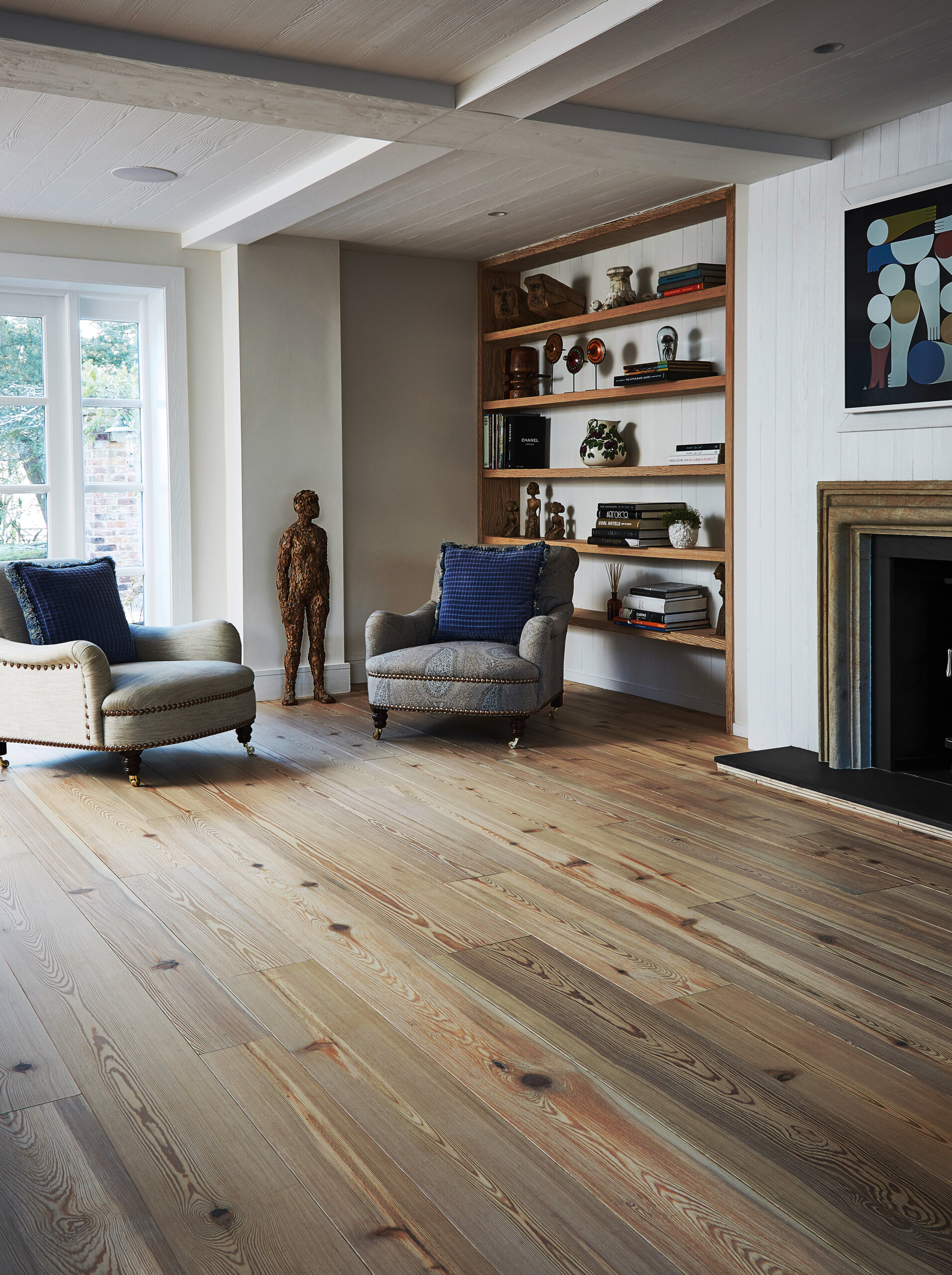# HOW TO MEASURE A ROOM FOR FLOORING

You need to understand the measurements of a room to know how much flooring will be needed to cover the desired area correctly. We have put together a quick how-to guide to help you with this. It is important to note that depending on the shape of your room, the method of measuring may differ.

When ordering the total amount of flooring you require, we recommend ordering a little over to allow for wastage and cutting, 5%-10% extra would be enough to account for any extra you may need.

## Equipment You Need

First things first, you the list below to accurately measure a room:

• A pen or pencil
• A tape measure
• A calculator or smart phone

## MEASURING A RECTANGLE ROOM

Measuring a rectangle is very straightforward. You will need to work out the area of the desired room. Simply take a tape measure and measure the length and width of the room. Note the measurements down and multiply the length by the width of the room together for the total area of the room. The resulting area is your required amount of flooring.

For example, if the length of your room is 8m and the width is 6m, the workings out would look like this: 8m x 6m = 48m

## MEASURING AN L-SHAPED ROOM

The easiest way of measuring an L-shaped room would be to separate the area into two rectangular sections. This means you can use the previous method we just discussed for an L-shaped room. Work out the area of the room by measuring the length and the width of each rectangle, multiplying the length by the width. Do this for both sections and add the resulting areas together for the final area of the whole room.

## MEASURING A COMPLEX SHAPED ROOM

If the room you are measuring is more complex, there’s no need to stress. Simply separate each section of the room into rectangles and follow the above method, adding the area of each section together for your final result.

Now you know how to measure your room for new flooring, we recommend reading our Wood Floor Installation Guide